Home
Hostname: page-component-55597f9d44-ssw5r Total loading time: 0.484 Render date: 2022-08-15T00:13:31.948Z Has data issue: true Feature Flags: { "shouldUseShareProductTool": true, "shouldUseHypothesis": true, "isUnsiloEnabled": true, "useRatesEcommerce": false, "useNewApi": true } hasContentIssue true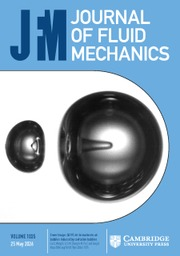Journal of Fluid Mechanics

## Abstract

Turbulent flows behind two side-by-side square cylinders with three different gap ratios, namely,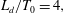$L_{d}/T_{0}=4,$ 6 and 8 (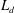$L_{d}$ is the separation distance between two cylinders and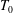$T_{0}$ is the cylinder thickness) are investigated by using direct numerical simulations. Depending on the strength of the gap flow, the three cases can generally be characterized into two regimes, one being the weak gap flow regime and the other being the robust gap flow regime. The wake-interaction length scale can only be applied to characterize the spatial evolution of the dual-wake flow in the robust gap flow regime. And only in this regime can the so-called ‘extreme events’ (i.e. non-Gaussian velocity fluctuations with large flatness) be identified. For the case with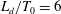$L_{d}/T_{0}=6$, two downstream locations, i.e.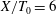$X/T_{0}=6$ and 26, at which the turbulent flows are highly non-Gaussian distributed and approximately Gaussian distributed, respectively, are analysed in detail. A well-defined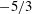$-5/3$ energy spectrum can be found in the near-field region (i.e.$X/T_{0}=6$), where the turbulent flow is still developing and highly intermittent and Kolmogorov’s universal equilibrium hypothesis does not hold. We confirm that the approximate$-5/3$ power law in the high-frequency range is closely related to the occurrences of the extreme events. As the downstream distance increases, the velocity fluctuations gradually adopt a Gaussian distribution, corresponding to a decrease in the strength of the extreme events. Consequently, the range of the$-5/3$ power law narrows. In the upstream region (i.e.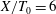$X/T_{0}=6$), the second-order structure function exhibits a power-law exponent close to$1$, whereas in the far downstream region (i.e.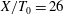$X/T_{0}=26$) the expected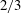$2/3$ power-law exponent appears. The larger exponent at$X/T_{0}=6$ is related to the fact that fluid motions in the intermediate range can directly ‘feel’ the large-scale vortex shedding.

## JFM classification

Type
JFM Papers
Information
Journal of Fluid Mechanics , 10 September 2019 , pp. 677 - 698

## Access options

Get access to the full version of this content by using one of the access options below. (Log in options will check for institutional or personal access. Content may require purchase if you do not have access.)

## References

Alam, M. M., Bai, H. L. & Zhou, Y. 2016 The wake of two staggered square cylinders. J. Fluid Mech. 801, 475507.CrossRefGoogle Scholar
Alam, M. M. & Zhou, Y. 2013 Intrinsic features of flow around two side-by-side square cylinders. Phys. Fluids 25, 085106.CrossRefGoogle Scholar
Alam, M. M., Zhou, Y. & Wang, X. W. 2011 The wake of two side-by-side square cylinders. J. Fluid Mech. 669, 432471.CrossRefGoogle Scholar
Frisch, U. 1995 Turbulence: The Legacy of A. N. Kolmogorov. Cambridge University Press.CrossRefGoogle Scholar
Gomes-Fernandes, R., Ganapathisubramani, B. & Vassilicos, J. C. 2012 Particle image velocimetry study of fractal-generated turbulence. J. Fluid Mech. 711, 306336.CrossRefGoogle Scholar
Gomes-Fernandes, R., Ganapathisubramani, B. & Vassilicos, J. C. 2014 Evolution of the velocity-gradient tensor in a spatially developing turbulent flow. J. Fluid Mech. 756, 252292.CrossRefGoogle Scholar
Hearst, R. J. & Lavoie, P. 2014 Scale-by-scale energy budget in fractal element grid-generated turbulence. J. Turbul. 15, 540554.CrossRefGoogle Scholar
Hurst, D. & Vassilicos, J. C. 2007 Scalings and decay of fractal-generated turbulence. Phys. Fluids 19, 035103.CrossRefGoogle Scholar
Isaza, J. C., Salazar, R. & Warhaft, Z. 2014 On grid-generated turbulence in the near- and far field regions. J. Fluid Mech. 753, 402426.CrossRefGoogle Scholar
Kolář, V., Lyn, D. A. & Rodi, W. 1997 Ensemble-averaged measurements in the turbulent near wake of two side-by-side square cylinders. J. Fluid Mech. 346, 201237.CrossRefGoogle Scholar
Kolmogorov, A. N. 1941a Dissipation of energy in the locally isotropic turbulence. Dokl. Akad. Nauk SSSR 32, 1618.Google Scholar
Kolmogorov, A. N. 1941b The local structure of turbulence in incompressible viscous fluid for very large Reynolds numbers. Dokl. Akad. Nauk SSSR 30, 301305.Google Scholar
Kolmogorov, A. N. 1941c On the degeneration of isotropic turbulence in an incompressible viscous fluid. Dokl. Akad. Nauk SSSR 31, 538540.Google Scholar
Kraichnan, R. H. 1974 On Kolmogorov’s inertial range theories. J. Fluid Mech. 62, 305330.CrossRefGoogle Scholar
Laizet, S. & Lamballais, E. 2009 High-order compact schemes for incompressible flows: a simple and efficient method with the quasi-spectral accuracy. J. Comput. Phys. 228, 59896015.CrossRefGoogle Scholar
Laizet, S., Lamballais, E. & Vassilicos, J. C. 2010 A numerical strategy to combine high-order schemes, complex geometry and parallel computing for high resolution dns of fractal generated turbulence. Comput. Fluids 39, 471484.CrossRefGoogle Scholar
Laizet, S. & Li, N. 2011 Incompact3d, a powerful tool to tackle turbulence problems with up to O (105) computational cores. Intl J. Numer. Meth. Fluids 67, 17351757.CrossRefGoogle Scholar
Laizet, S., Nedić, J. & Vassilicos, J. C. 2015a Influence of the spatial resolution on fine-scale features in dns of turbulence generated by a single square grid. Intl J. Comput. Fluid Dyn. 29, 286302.CrossRefGoogle Scholar
Laizet, S., Nedić, J. & Vassilicos, J. C. 2015b The spatial origin of - 5/3 spectra in grid-generated turbulence. Phys. Fluids 27, 065115.CrossRefGoogle Scholar
Laizet, S., Vassilicos, J. C. & Cambon, C. 2013 Interscale energy transfer in decaying turbulence and vorticity-strain-rate dynamics in grid-generated turbulence. Fluid Dyn. Res. 45, 061408.Google Scholar
Lele, S. K. 1992 Compact finite difference schemes with spectral-like resolution. J. Comput. Phys. 103, 1642.CrossRefGoogle Scholar
Mazellier, N. & Vassilicos, J. C. 2010 Turbulence without Richardson–Kolmogorov cascade. Phys. Fluids 22, 075101.CrossRefGoogle Scholar
Nagata, K., Saiki, T., Sakai, Y., Ito, Y. & Iwano, K. 2017 Effects of grid geometry on non-equilibrium dissipation in grid turbulence. Phys. Fluids 29, 015102.CrossRefGoogle Scholar
Nagata, K., Sakai, Y., Inaba, T., Suzuki, H., Terashima, O. & Suzuki, H. 2013 Turbulence structure and turbulence kinetic energy transport in multiscale/fractal-generated turbulence. Phys. Fluids 25, 065102.CrossRefGoogle Scholar
Parnaudeau, P., Carlier, J., Heitz, D. & Lamballais, E. 2008 Experimental and numerical studies of the flow over a circular cylinder at Reynolds number 3900. Phys. Fluids 20, 085101.CrossRefGoogle Scholar
Paul, I., Papadakis, G. & Vassilicos, J. C. 2017 Genesis and evolution of velocity gradients in near-field spatially developing turbulence. J. Fluid Mech. 815, 295332.CrossRefGoogle Scholar
Paul, I., Papadakis, G. & Vassilicos, J. C. 2018 Evolution of passive scalar statistics in a spatially developing turbulence. Phys. Rev. Fluids 3, 014612.CrossRefGoogle Scholar
Pope, S. B. 2000 Turbulent Flows. Cambridge University Press.10.1017/CBO9780511840531CrossRefGoogle Scholar
Portela, F. A., Papadakis, G. & Vassilicos, J. C. 2017 The turbulence cascade in the near wake of a square prism. J. Fluid Mech. 825, 315352.CrossRefGoogle Scholar
Redford, J. A., Castro, I. P. & Coleman, G. N. 2012 On the universality of turbulent axisymmetric wakes. J. Fluid Mech. 710, 419452.CrossRefGoogle Scholar
da Silva, C. B., Hunt, J. C. R., Eames, I. & Westerweel, J. 2014 Interfacial layers between regions of different turbulence intensity. Annu. Rev. Fluid Mech. 46, 567590.CrossRefGoogle Scholar
Tennekes, H. & Lumley, J. L. 1972 A First Course in Turbulence. MIT Press.CrossRefGoogle Scholar
Townsend, A. A. 1956 The Structure of Turbulent Shear Flows. Cambridge University Press.Google Scholar
Trias, F. X., Gorobets, A. & Oliva, A. 2015 Turbulent flow around a square cylinder at Reynolds number 22,000: a DNS study. J. Fluid Mech. 123, 8798.Google Scholar
Valente, P. C. & Vassilicos, J. C. 2011 The decay of turbulence generated by a class of multiscale grids. J. Fluid Mech. 687, 300340.CrossRefGoogle Scholar
Williamson, C. H. K. 1996 Vortex dynamics in the cylinder wake. Annu. Rev. Fluid Mech. 28, 477539.CrossRefGoogle Scholar
Zheng, Q. & Alam, M. M. 2017 Intrinsic features of flow past three square prisms in side-by-side arrangement. J. Fluid Mech. 826, 9961033.CrossRefGoogle Scholar
Zhou, Y., Nagata, K., Sakai, Y., Ito, Y. & Hayase, T. 2015 On the evolution of the invariants of the velocity gradient tensor in single-square-grid-generated turbulence. Phys. Fluids 27, 075107.CrossRefGoogle Scholar
Zhou, Y., Nagata, K., Sakai, Y., Ito, Y. & Hayase, T. 2016a Enstrophy production and dissipation in developing grid-generated turbulence. Phys. Fluids 28, 025113.CrossRefGoogle Scholar
Zhou, Y., Nagata, K., Sakai, Y., Ito, Y. & Hayase, T. 2016b Spatial evolution of the helical behavior and the 2/3 power-law in single-square-grid-generated turbulence. Fluid Dyn. Res. 48, 021404.Google Scholar
Zhou, Y., Nagata, K., Sakai, Y., Suzuki, H., Ito, Y., Terashima, O. & Hayase, T. 2014a Development of turbulence behind the single square grid. Phys. Fluids 26, 045102.CrossRefGoogle Scholar
Zhou, Y., Nagata, K., Sakai, Y., Suzuki, H., Ito, Y., Terashima, O. & Hayase, T. 2014b Relevance of turbulence behind the single square grid to turbulence generated by regular- and multiscale-grids. Phys. Fluids 26, 075105.CrossRefGoogle Scholar
Zhou, Y., Nagata, K., Sakai, Y. & Watanabe, T. 2018 Dual-plane turbulent jets and their non-Gaussian velocity fluctuations. Phys. Rev. Fluids 3, 124604.CrossRefGoogle Scholar
Zhou, Y., Wang, Z. J., Xu, S. J. & Jin, W. 2001 Free vibrations of two side-by-side cylinders in a cross-flow. J. Fluid Mech. 443, 197229.CrossRefGoogle Scholar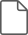### Zhou et al. supplementary material

Zhou et al. supplementary material 1

File 433 KB
8
Cited by

# Save article to Kindle

Note you can select to save to either the @free.kindle.com or @kindle.com variations. ‘@free.kindle.com’ emails are free but can only be saved to your device when it is connected to wi-fi. ‘@kindle.com’ emails can be delivered even when you are not connected to wi-fi, but note that service fees apply.

Find out more about the Kindle Personal Document Service.

Extreme events and non-Kolmogorov$-5/3$spectra in turbulent flows behind two side-by-side square cylinders Available formats × # Save article to Dropbox To save this article to your Dropbox account, please select one or more formats and confirm that you agree to abide by our usage policies. If this is the first time you used this feature, you will be asked to authorise Cambridge Core to connect with your Dropbox account. Find out more about saving content to Dropbox. Extreme events and non-Kolmogorov$-5/3$ spectra in turbulent flows behind two side-by-side square cylinders
Available formats
×

# Save article to Google Drive

To save this article to your Google Drive account, please select one or more formats and confirm that you agree to abide by our usage policies. If this is the first time you used this feature, you will be asked to authorise Cambridge Core to connect with your Google Drive account. Find out more about saving content to Google Drive.

Extreme events and non-Kolmogorov$-5/3\$ spectra in turbulent flows behind two side-by-side square cylinders
Available formats
×
×